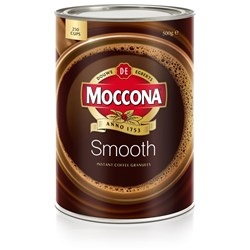Select Office Supplies

# Food & Beverages

\$13.62 \$14.99 inc GST ex GST (EACH)
Increase value Decrease value
\$3.40 \$3.75 inc GST ex GST (EACH)
Increase value Decrease value
\$3.40 \$3.75 inc GST ex GST (EACH)
Increase value Decrease value
\$21.77 \$23.95 inc GST ex GST (EACH)
Increase value Decrease value
\$4.40 \$4.85 inc GST ex GST (PACK)
Increase value Decrease value
\$5.13 \$5.65 inc GST ex GST (PACK)
Increase value Decrease value
\$8.59 \$9.45 inc GST ex GST (EACH)
Increase value Decrease value
\$6.68 \$7.35 inc GST ex GST (EACH)
Increase value Decrease value
\$6.13 \$6.75 inc GST ex GST (EACH)
Increase value Decrease value
\$13.54 \$14.90 inc GST ex GST (EACH)
Increase value Decrease value
\$9.90 \$10.90 inc GST ex GST (EACH)
Increase value Decrease value
\$9.77 \$10.75 inc GST ex GST (EACH)
Increase value Decrease value
\$12.63 \$13.90 inc GST ex GST (EACH)
Increase value Decrease value
\$62.99 \$62.99 inc GST ex GST (EACH)
Increase value Decrease value
\$46.99 \$46.99 inc GST ex GST (EACH)
Increase value Decrease value
\$81.77 \$89.94 inc GST ex GST (EACH)
Increase value Decrease value
\$90.86 \$99.94 inc GST ex GST (EACH)
Increase value Decrease value
\$90.86 \$99.94 inc GST ex GST (EACH)
Increase value Decrease value
\$118.14 \$129.95 inc GST ex GST (EACH)
Increase value Decrease value
\$136.31 \$149.94 inc GST ex GST (EACH)
Increase value Decrease value
\$118.14 \$129.95 inc GST ex GST (EACH)
Increase value Decrease value
\$17.22 \$18.95 inc GST ex GST (EACH)
Increase value Decrease value
\$58.90 \$58.90 inc GST ex GST (EACH)
Increase value Decrease value
\$23.59 \$23.59 inc GST ex GST (EACH)
Increase value Decrease value
\$79.99 \$79.99 inc GST ex GST (EACH)
Increase value Decrease value
\$38.00 \$38.00 inc GST ex GST (PACK)
Increase value Decrease value
\$165.00 \$165.00 inc GST ex GST (EACH)
Increase value Decrease value
\$59.99 \$59.99 inc GST ex GST (TIN)
Increase value Decrease value
\$42.75 \$42.75 inc GST ex GST (TIN)
Increase value Decrease value
\$39.00 \$39.00 inc GST ex GST (TIN)
Increase value Decrease value\$31.99 \$31.99 inc GST ex GST (EACH)
Increase value Decrease value
\$7.49 \$7.49 inc GST ex GST (EACH)
Increase value Decrease value
\$53.59 \$53.59 inc GST ex GST (BOX)
Increase value Decrease value
\$47.99 \$47.99 inc GST ex GST (EACH)
Increase value Decrease value
\$39.99 \$39.99 inc GST ex GST (TIN)
Increase value Decrease value
\$44.54 \$44.54 inc GST ex GST (EACH)
Increase value Decrease value
##### Products to compare:
Comparing Products